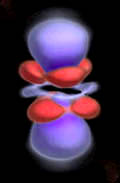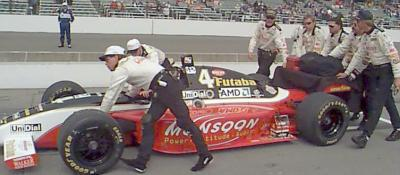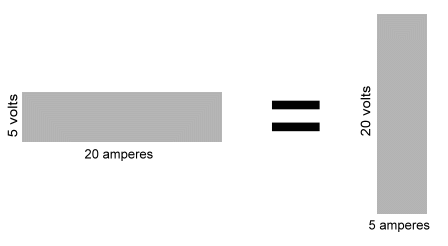## Electricity

What we call electricity is the effect of tiny things that carry electric charge. Electric charge comes in two forms positive and negative.

Opposites attract.
Positive charges are attracted to negative charges.
Negative charges are attracted to positive charges.

Positive charges repel other positive charges.
Negative charges repel other negative charges.

In this way, charges act somewhat like magnets.

Inside every atom are protons and electrons. Protons are positively charged. Electrons are negatively charged.

Protons are many times heavier than the electrons, and they group together in the nucleus of the atom. They are stuck together with a force called the strong force. It is called strong because it is much stronger than the repulsion that the protons feel towards one another.

The electrons do not interact with the strong force, so they don't clump together like the protons do. They are attracted to the protons, so they stay as close to the nucleus as they can. The rules of quantum mechanics limit how many electrons can occupy the spaces close to the nucleus, so the electrons arrange themselves in clouds at different distances (different energy levels) from the nucleus.The locations where an electron is most likely to be found take the form of spheres, doughnuts, dumbells, and petals around the central nucleus of this copper atom.

## Electrons

Since the protons are heavy and clump together in the nucleus, they tend to stay put. Thus the particle that we usually find carrying electric charge from place to place is the electron.

Electrons are small and light, and move about easily. Since they are all the same charge, they repel one another.

In some materials, the atoms bind tightly to their electrons. In other materials, the electrons are more loosly bound. As the spaces close to the positively charged nucleus get filled with electrons, the remaining electrons fill shells farther and farther from the nucleus. These electrons are easier to remove from the atom than the electrons closer to the nucleus. Just like magnets, the forces attracting electrons to protons get weaker with distance.

If we touch together two different materials, such as a plastic comb and a cat's fur coat, one material will attract electrons from the other. The material with more electrons (the comb) now has a negative charge, leaving the other material (the fur) with a positive charge. They will attract one another because they have different charges. As you hold the comb a little bit above the cat, the cat's fur will rise to meet the comb.

By rubbing a lot of electrons onto the comb, we can use the electrons to do work.

Since electrons repel one another, they will push each other off of the comb if they have somewhere to go. If you touch the comb to the wet nose of the cat, the electrons will jump back to the cat with an audible snap and a little spark of light. The sound energy and the light energy were created by moving electrons. (Other effects are caused by the now moving cat).

## Electric power

Since electrons repel one another, as we crowd them together onto something, it gets harder and harder to put each electron in with the others. But when we finally let them out, the energy we used to cram them together is released like a spring, and we can use the energy to do useful work.

There are two things that determine how much energy we have. One is simply how many electrons there are. The other is how closely they are crowded together.

Since electrons are extremely small, we need extremely large numbers of them to do useful things. If we have 6,240,000,000,000,000,000 of them (6.24 quintillion) we have a coulomb of electrons.

To measure how tightly packed the electrons are, we look at how quickly they move when we let them out. If the electrons are squeezed tightly together, they will push harder against one another, and so they will move faster when they are let go, just like a spring, or the air in a rubber balloon.

The force required to move one coulomb of electrons in one second is called a volt. Voltage is the pressure of electrons pushing against one another.

When electrons are moving, we have a current. Current is measured by how many electrons move past a point in one second. If one coulomb of electrons moves past a point in one second, we say we have one ampere of current.

If you wanted to push a car that was out of gas, you could use one person pushing very hard, or you could use a bunch of people pushing lightly. The power to move the car is the same either way. With electrons, we can also use a few pushing very hard, or we could use many, pushing lightly.In electrical terms, how hard you push is the voltage. How many cars get pushed into the gas station per hour is the current.

The word work is used to describe using a force to move something. Moving a car is work. One person pushing hard can move a car slowly. Two people pushing hard can move the car twice as fast.

How fast work is done is called power.

With electricity, power is the voltage multiplied by the current. Power is measured in watts. A watt is one volt times one ampere. An older unit of power is the horsepower. One horsepower is 745.7 watts. A tractor with more horsepower can do more work per hour than one with less horsepower.

If you have 100 watts, you can do 10 times as much work per second as you can if you only have 10 watts.

If we have 5 volts of pressure pushing 20 coulombs of electrons past a point in one second, we have 100 watts of power. But we could also get the same power by having 20 volts pushing 5 coulombs of electrons past a point in a second.Power is often measured in 1,000 watt units called kilowatts. A kilowatt is 1.34 horsepower. Sometimes electric motors are rated by their horsepower. A 3/4 horsepower motor is the same as a 560 watt motor. A motor like that, working as hard as it can, will use 0.56 killowatts of power.

Electric companies charge by the kilowatt hour. If you run a 560 watt motor as hard as it can for an hour and 47 minutes, you will be charged for 1 kilowatt hour of electricity. That's the same charge you would pay to run a 100 watt light bulb for 10 hours.Send mail to Simon Quellen Field via sfield@scitoys.com

>